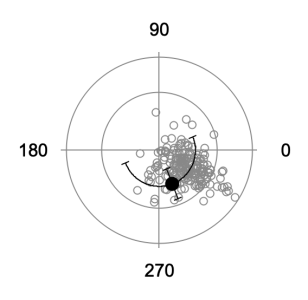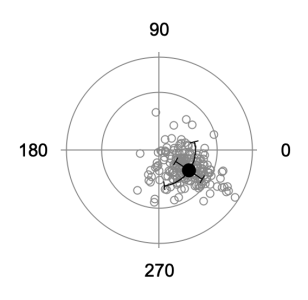# Polar averaging and errors

Averaging waves with polar data (list of angles) will give incorrect results in many cases (see image below). This function does a vector summation of X and Y components to determine the average angle, the standard deviation, or the standard error.

Wave inWave //single wave with list of angles

//opStr = "avg", "sdev", or "sem"
//degrad = "deg" or "rad" depending on the input data type
Variable i,size,avgX,avgY,avg,sdev,sem,errX,errY,value,multiplier

size = DimSize(inWave,0)
Make/FREE/N=(size) xTotal,yTotal

//input is degrees or radians
case "deg":
xTotal = cos(inWave * pi/180)
yTotal = sin(inWave * pi/180)
multiplier = 180/pi
break
xTotal = cos(inWave)
yTotal = sin(inWave)
multiplier = 1
break
endswitch

//polar average
avgX = mean(xTotal)
avgY = mean(yTotal)
avg = atan2(avgY,avgX)*multiplier

If(avg < 0)
avg += 360
EndIf

//polar standard deviation
For(i=0;i<size;i+=1)
errX += (xTotal[i] - avgX)^2/(size-1)
errY += (yTotal[i] - avgY)^2/(size-1)
EndFor

errX = sqrt(errX)
errY = sqrt(errY)
sdev = atan2(errY,errX)*multiplier

//polar standard error of the mean
sem = sdev/sqrt(size)

//select return parameter
strswitch(opStr)
case "avg":
value = avg
break
case "sdev":
value = sdev
break
case "sem":
value = sem
break
endswitch

return value
EndI find the description and polar plots a little confusing. You imply that the only input is a 1-D wave of polar angles (or you use only the angular part of a {theta, r} wave), but the data set has multiple 'r' values. Are you selecting a constant-r set of values from your presented data?

Another approach for full 2-D (x,y) or (r,theta)-->(x,y) data might be to first perform a linear ODR fit to an (x,y) line constrained to pass through the origin to find the best-fit average angle. Then find the difference angle for each point to get the set of d_theta's for the standard deviation calculation. I note that StatsCircularMoments seems to apply only to 1-D data at a fixed radius.ForumSupportGallery